Equivalent Fractions

In the produced worksheet, kids will solve simple problems where equivalent fractions are rendered to a sheet with either Numerator or Denominator left blank. A starter fraction will be provided.

Ordering

 Smallest numerator to largest numerator.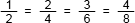Any order.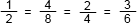Gaps

 Gaps in the list of fractions are not allowedGaps in the list of fractions are allowed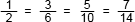Show Lowest Terms Fraction

 Always show the lowest terms fraction complete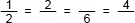Show any fraction in the list complete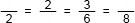Number of Worksheets